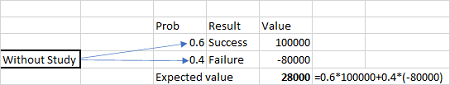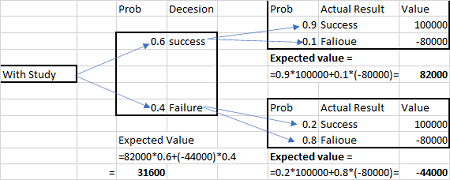# Sue Reynolds is considering investing in a retail store. Sue estimates that the probability of a...

## Question:

Sue Reynolds is considering investing in a retail store. Sue estimates that the probability of a successful store will be 0.6. A successful store will give a return of $100,000. If Sue invests in the store but it is unsuccessful, she will see a loss of$80,000.

a). Sue has the option of conducting a study (at a cost of $20,000) as input into her investment decision. If she conducts the study, there is a 0.6 probability that the study will predict the store will be successful and a 0.4 probability that the study will predict the store will be unsuccessful (these values are the marginal probabilities). If the study predicts the store will be successful, there is a 0.9 probability that the store will be a success yet if the study predicts the store will be unsuccessful the probability the store is unsuccessful is 0.8 (these values are posterior probabilities). Formulate a two-stage decision tree to capture both the obtain information decision and the build the store decision. Use the Tree Plan to construct and solve a decision tree for this problem. What are Sue's optimal decisions with the EMV criteria? b) What is the expected net gain from sampling? ## Decision Tree: A decision tree is a tree-shaped pictorial view of the decision mechanism which is used to finalize the correct alternative among multiple alternatives. In such a case, each alternative is attached with defined probabilities and the expected value is calculated from the probable options. ## Answer and Explanation: Become a Study.com member to unlock this answer! Create your account (a) What are Sue's optimal decisions with the EMV criteria? EMV without study:EMV with study:EMV =$31600 - \$20,000 =...What Is a Decision Tree? - Examples, Advantages & Role in Management

from

Chapter 2 / Lesson 12
61K

Clearly identifying all possible solutions for a given decision is an important part of successful management. In this lesson, you will learn how to use a decision tree to identify and select possible courses of action.